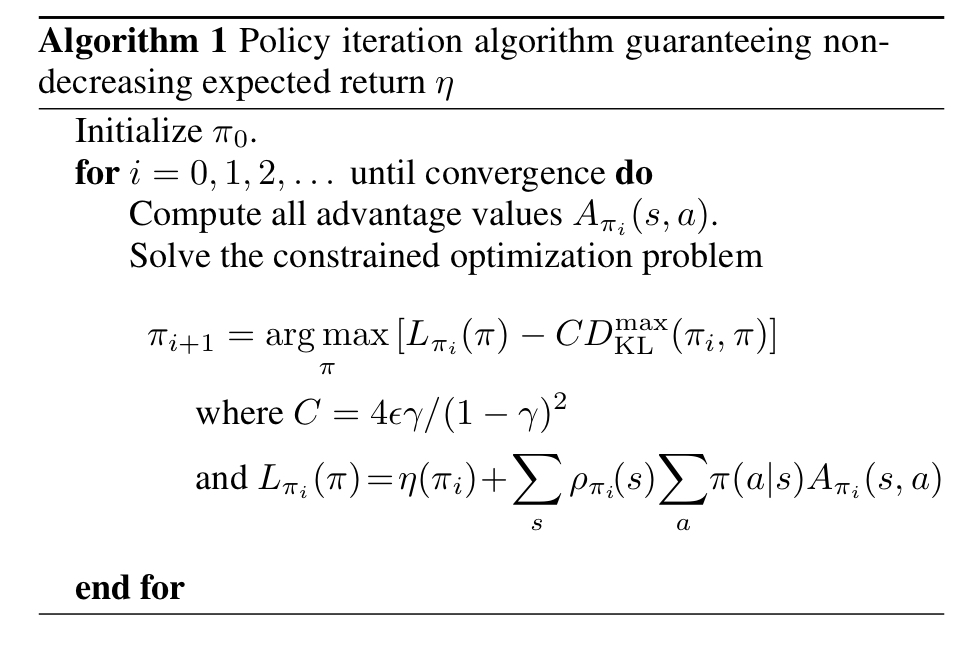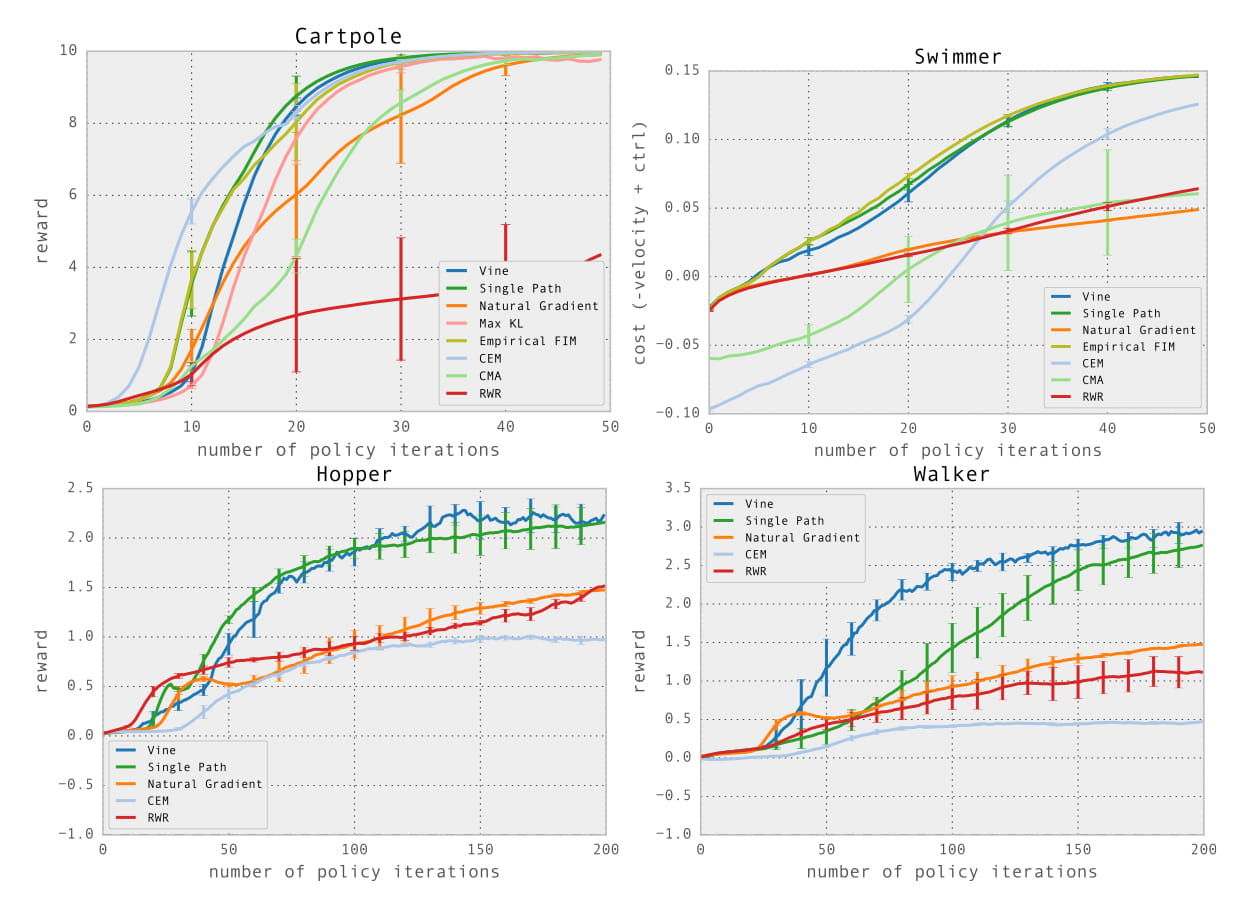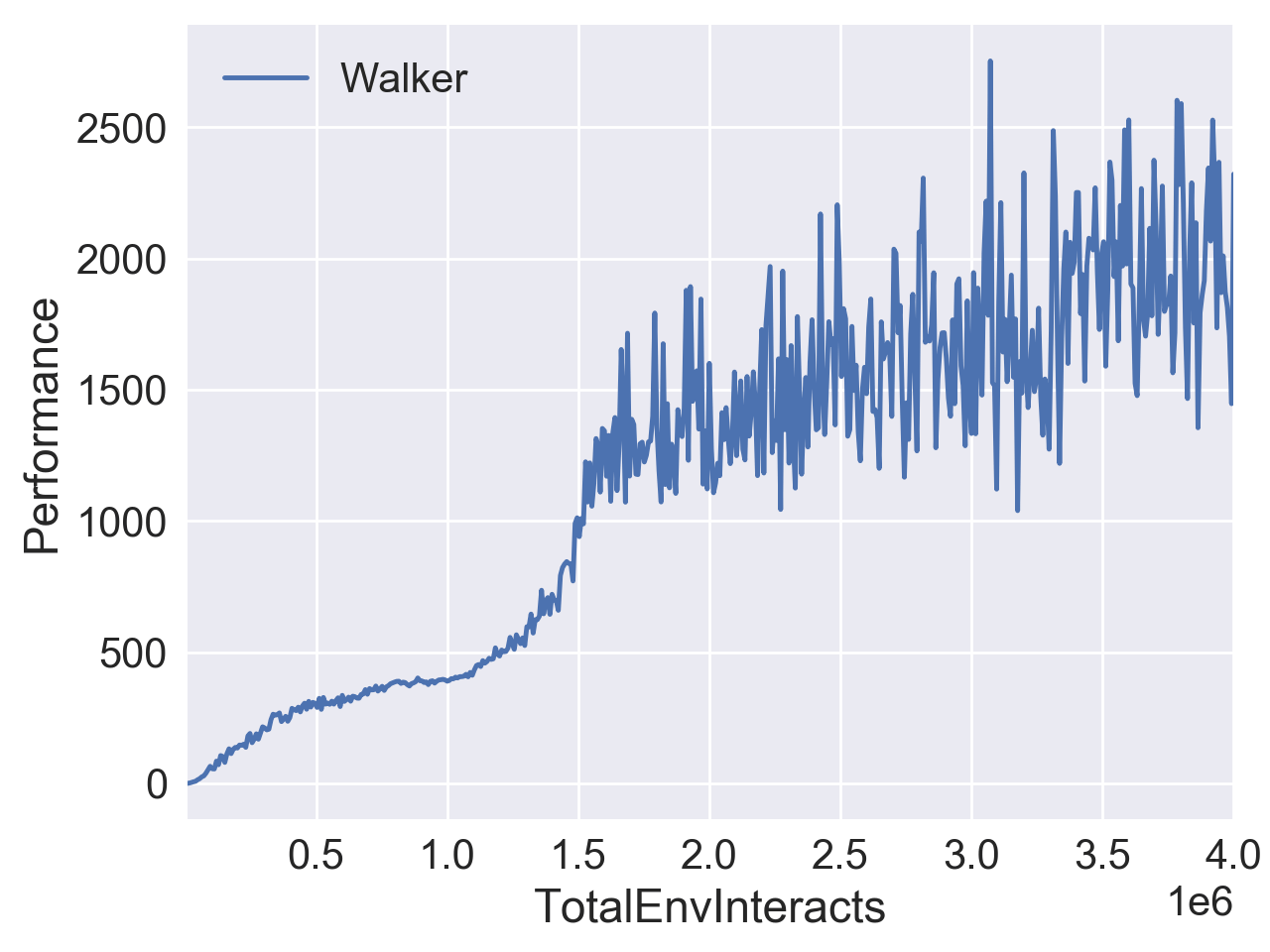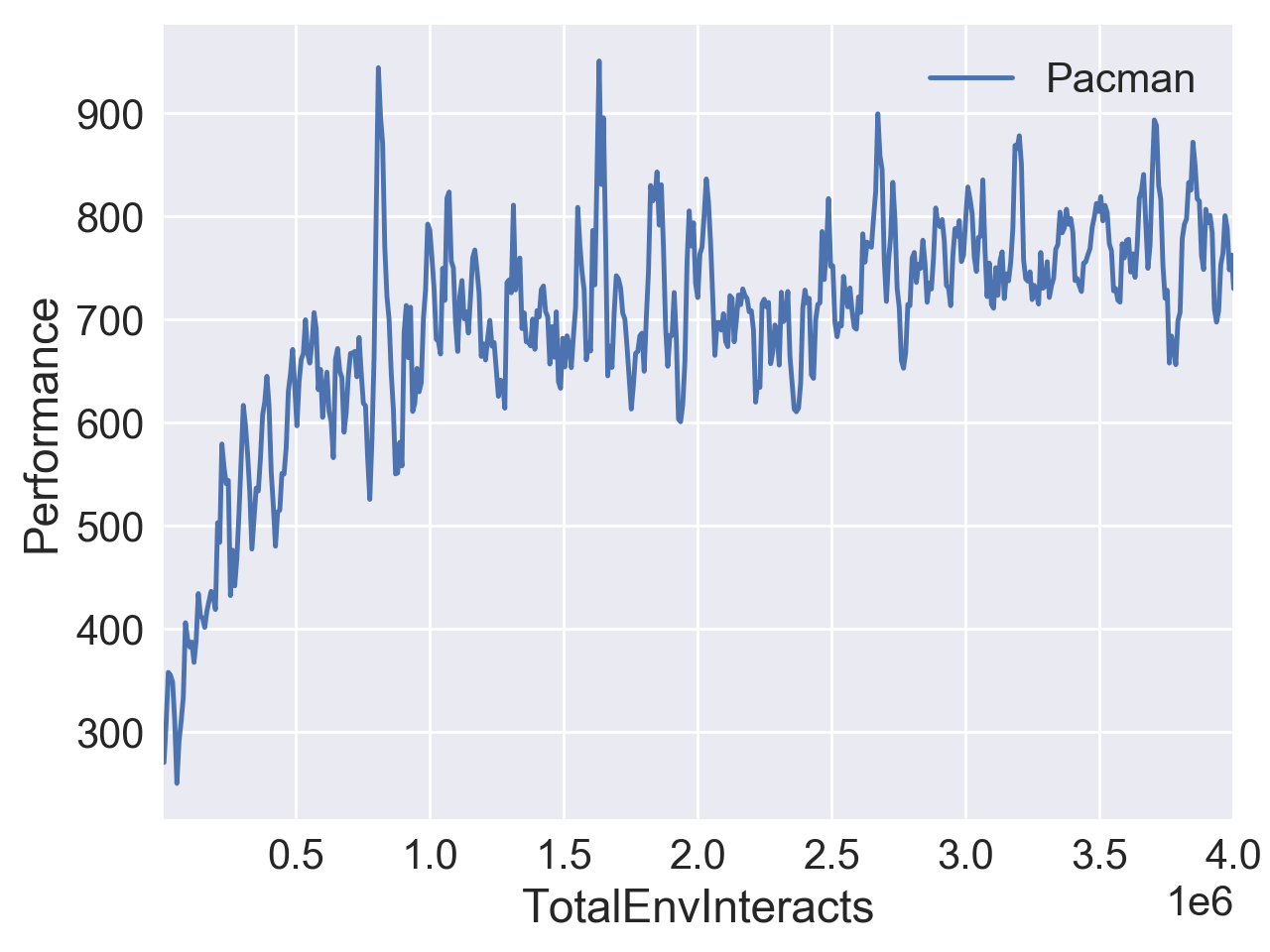# NIUHE

### RL - Trust Region Policy Optimization (TRPO)

Trust Region Policy Optimization (TRPO)算法是由伯克利大学的Schulman等人于2015年提出的策略梯度（Policy Gradients）算法。TRPO通过最大化新策略相对于当前策略的优势来保证每次更新都是单调递增的（稳定），同时找到尽可能大的更新步幅。算法推导出的最终结果是在KL约束下最大化替代优势函数。

## 背景

$V_\pi(s_t) =\mathbb{E}_{a_t, s_{t+1}, ...\sim\pi}[\sum_{l=0}^\infty\gamma^lr_{s_{t+l}}]$

$A_\pi(s, a) = Q_\pi(s, a) - V_\pi(s)$## TRPO

$\bar{D}_{KL}(\theta||\theta_{old})\approx \frac{1}{2}(\theta-\theta_{old})^TH(\theta-\theta_{old})$

$H\hat{x} = \triangledown_\theta((\triangledown_\theta\bar{D}_{KL}(\theta||\theta_k))^T\hat{x})$

TRPO算法## Performance

TRPO的一些特点

• 保证每次更新在当前训练数据上都是进步的，训练过程更加稳定
• 通过满足KL约束来找尽可能大的步长
• on-policy 算法
• 可用于离散和连续的动作空间
• 算法较为复杂